cdiff## cdiff

بازدید : 541 شنبه 20 دي 1393

## cdiff

`cdiff(expr,dim)`

Performs a centered difference operation on `expr` in the direction specified by `dim`. The difference is done in the grid space, and no adjustment is performed for unequally spaced grids. The result value at each grid point is the value at the grid point plus one minus the value at the grid point minus one. The `dim` argument specifies the dimension over which the difference is to be taken, and is a single character: X, Y, Z, or T.

Result values at the grid boundaries are set to missing.

### Examples

1. The `cdiff` function may be used to duplicate the calculation done by the `hcurl` function:

`define dv = cdiff(v,x)`
`define dx = cdiff(lon,x)*3.1416/180`
`define du = cdiff(u*cos(lat*3.1416/180),y)`
`define dy = cdiff(lat,y)*3.1416/180`
display (dv/dx-du/dy)/(6.37e6*cos(lat*3.1416/180))

The above example assumes an X-Y varying dimension environment. Note that the intrinsic variables `lat` and `lon` give results in degrees and must be converted to radians in the calaculation. Also note the radius of the earth is assumed to be `6.37e6` meters thus the U and V winds are assumed to have units of `m/sec`.

2. Temperature advection can be calculated using the `cdiff` function as follows:

`define dtx = cdiff(t,x)`
`define dty = cdiff(t,y)`
`define dx = cdiff(lon,x)*3.1416/180`
`define dy = cdiff(lat,y)*3.1416/180`
display -1*( (u*dtx)/(cos(lat*3.1416/180)*dx) + v*dty/dy )/6.37e6

where the variable `t` is temperature, `u` is the U component of the wind, and `v` is the V component of the wind.

مطالب مرتبط
ارسال نظر برای این مطلب

نام
ایمیل (منتشر نمی‌شود) (لازم)
وبسایت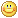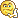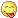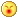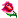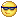نظر خصوصی
مشخصات شما ذخیره شود ؟ [حذف مشخصات] [شکلک ها]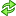کد امنیتی
اطلاعات کاربری
 نام کاربری : رمز عبور :
• فراموشی رمز عبور؟
• لینک دوستان
آرشیو
آمار سایت
• کل مطالب : 155
• کل نظرات : 0
• افراد آنلاین : 1
• تعداد اعضا : 4
• آی پی امروز : 5
• آی پی دیروز : 31
• بازدید امروز : 58
• باردید دیروز : 55
• گوگل امروز : 0
• گوگل دیروز : 0
• بازدید هفته : 251
• بازدید ماه : 326
• بازدید سال : 27,260
• بازدید کلی : 148,312
• کدهای اختصاصی